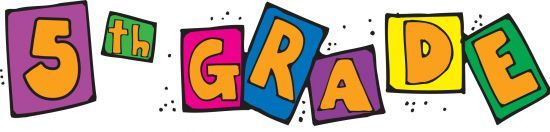In 5th grade math, instructional time should focus on three critical areas: (1) developing fluency with addition and subtraction of fractions, and developing understanding of the multiplication of fractions and of division of fractions in limited cases (unit fractions divided by whole numbers and whole numbers divided by unit fractions); (2) extending division to 2-digit divisors, integrating decimal fractions into the place value system and developing understanding of operations with decimals to hundredths, and developing fluency with whole number and decimal operations; and (3) developing understanding of volume.